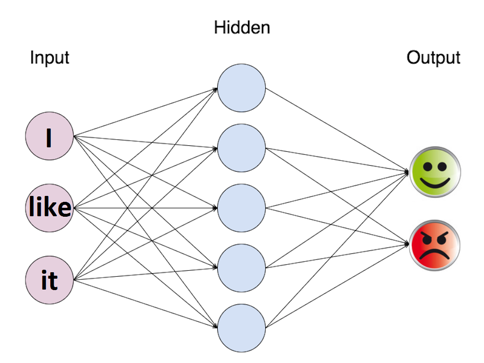# Sentiment analysis Analysis Part 3  – Neural Networks

In the next set of topics we will dive into different approachs to solve the hello world problem of the NLP world, the sentiment analysis.

Check the other parts: Part1 Part2 Part3

The code for this implementation is at https://github.com/iolucas/nlpython/blob/master/blog/sentiment-analysis-analysis/neural-networks.ipynbSentiment analysis is an area of research that aims to tell if the sentiment of a portion of text is positive or negative.

#### The Code

We will use two machine learning libraries:

• scikit-learn to create onehot vectors from our text and split the dataset into train, test and validation;
• tensorflow to create the neural network and train it.

Our dataset is composed of movie reviews and labels telling whether the review is negative or positive. Let’s load the dataset:

The reviews file is a little big, so it is in zip format. Let’s Extract it with the the `zipfile` module:

```import zipfile
with zipfile.ZipFile("reviews.zip", 'r') as zip_ref:
zip_ref.extractall(".")```

Now that we have the reviews.txt and labels.txt files, we load them to the memory:

```with open("reviews.txt") as f:
with open("labels.txt") as f:

reviews_tokens = [review.split() for review in reviews]```

Next we load the module to transform our review inputs into binary vectors with the help of the class `MultiLabelBinarizer`:

```from sklearn.preprocessing import MultiLabelBinarizer

onehot_enc = MultiLabelBinarizer()
onehot_enc.fit(reviews_tokens)```

After that we split the data into training and test set with the `train_test_split `function. We then split the test set in half to generate a validation set:

```from sklearn.model_selection import train_test_split

X_train, X_test, y_train, y_test = train_test_split(reviews_tokens, labels, test_size=0.4, random_state=None)

split_point = int(len(X_test)/2)
X_valid, y_valid = X_test[split_point:], y_test[split_point:]
X_test, y_test = X_test[:split_point], y_test[:split_point]```

We then define two functions: label2bool, to convert the string label to a binary vector of two elements and get_batch, that is a generator to return parts of the dataset in a iteration:

```def label2bool(labels):
return [[1,0] if label == "positive" else [0,1] for label in labels]

def get_batch(X, y, batch_size):
for batch_pos in range(0,len(X),batch_size):
yield X[batch_pos:batch_pos+batch_size], y[batch_pos:batch_pos+batch_size] ```

Tensorflow connects expressions in structures called graphs. We first clear any existing graph , then get the vocabulary length and declare placeholders that will be used to input our text data and labels:

```tf.reset_default_graph()

vocab_len = len(onehot_enc.classes_)
inputs_ = tf.placeholder(dtype=tf.float32, shape=[None, vocab_len], name="inputs")
targets_ = tf.placeholder(dtype=tf.float32, shape=[None, 2], name="targets")```

This post does not intend to be a tensorflow tutorial, for more details visit https://www.tensorflow.org/get_started/

We then create our neural network:

• h1 is the hidden layer that received as input the text words vectors;
• logits is the final layer that receives the h1 as input;
• output is the result of applying the sigmoid function to the logits;
• loss is the loss expression to calculate the current error of the neural network;
• optimizer is the expression to adjust the weights of the neural network in order to reduce the loss expression;
• correct_pred and accuracy are used to calculate the current accuracy of the neural network ranging from 0 to 1.
```h1 = tf.layers.dense(inputs_, 500, activation=tf.nn.relu)
logits = tf.layers.dense(h1, 2, activation=None)
output = tf.nn.sigmoid(logits)

loss = tf.reduce_mean(tf.nn.softmax_cross_entropy_with_logits(logits=logits, labels=targets_))

correct_pred = tf.equal(tf.argmax(logits, 1), tf.argmax(targets_, 1))
accuracy = tf.reduce_mean(tf.cast(correct_pred, tf.float32), name='accuracy')```

We then train the network, periodically printing its current accuracy and loss:

```epochs = 10
batch_size = 3000

sess = tf.Session()

# Initializing the variables
sess.run(tf.global_variables_initializer())
for epoch in range(epochs):
for X_batch, y_batch in get_batch(onehot_enc.transform(X_train), label2bool(y_train), batch_size):
loss_value, _ = sess.run([loss, optimizer], feed_dict={
inputs_: X_batch,
targets_: y_batch
})
print("Epoch: {} \t Training loss: {}".format(epoch, loss_value))

acc = sess.run(accuracy, feed_dict={
inputs_: onehot_enc.transform(X_valid),
targets_: label2bool(y_valid)
})

print("Epoch: {} \t Validation Accuracy: {}".format(epoch, acc))

test_acc = sess.run(accuracy, feed_dict={
inputs_: onehot_enc.transform(X_test),
targets_: label2bool(y_test)
})
print("Test Accuracy: {}".format(test_acc))```

With this network we got an accuracy of 90%! With more data and using a bigger network we can improve this result even further!

#### Leave any questions and comments below

1.Lucas Oliveira says:

quase

2.Like says:

ZFN Liker, Status Auto Liker, Increase Likes, auto like, autolike, Autolike, Auto Like, Auto Liker, autoliker, Autolike International, Photo Auto Liker, Autoliker, Status Liker, auto liker, Working Auto Liker, Photo Liker, Autoliker

3.ปั้มไลค์ says:

Like!! Really appreciate you sharing this blog post.Really thank you! Keep writing.

4.ปั้มไลค์ says:

Like!! Great article post.Really thank you! Really Cool.

5.Mary Mcgraff says:

Would you be concerned with exchanging hyperlinks?

6.https://cbd-campus.com/ says:

Needed to send you a little bit of note to help thank you very much again for these superb tips you have shown in this article. It is simply incredibly generous of you to grant unhampered all that numerous people could have distributed for an e book to get some money for their own end, particularly since you might have done it in case you decided. These secrets additionally worked to be the great way to fully grasp that the rest have the same eagerness much like my own to learn a great deal more pertaining to this issue. I’m certain there are many more fun instances up front for individuals that looked over your site.

7.vurtilopmer says:

8.http://cbdadverts.com/ says:
9.http://cbdadverts.com/ says:
10.Chassidy Delcarmen says: